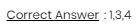# A confusing question on capacitors and voltages across them

• Gourab_chill

#### Gourab_chill

Homework Statement
I have posted the question as a attachment below. Please open the attachment to find the question.
Relevant Equations
Q=CV, Kirchoff's voltage law
In my opinion, the voltage across the C1 should be 9V as the potential on the side of the positive plate of the capacitor should be (15-6)V and on the other be 0V.
Similarly the potential across C2 should be (7-0)V.
Here I'm basically assuming that the voltage at the negative terminals of the cells to be zero, which is the point I'm mistaking probably. The answer to the question is-Please tell how to approach this question. Any help will be deeply appreciated.

#### Attachments

Here I'm basically assuming that the voltage at the negative terminals of the cells to be zero, which is the point I'm mistaking probably.
Yes, I beileve there's the mistake. You can assume only one point in the circuit to be at 0V (ANY one point).

You have a small loop formed by the two series capacitors and the 3 ohm resistor. Call the left plate of C1 as point p.
Consider this loop p-a-b-p to be your KVL loop and assume potential at point b to be 0.

What is the voltage across the 3 ohm resistor (VPB)?

•Delta2
Consider this loop p-a-b-p to be your KVL loop and assume potential at point b to be 0.

What is the voltage across the 3 ohm resistor (VPB)?

Well since the current flowing through the other circuit of which 3 ohm is common is 3A so the voltage across the resistance is 3*3=9V which I figured but the real problem I'm having is what is the voltage across the capacitors, how to apply KVL exactly- I'm having confusions regarding the charge on the capacitors too (which i think will be necessary for applying KVL?)

which i think will be necessary for applying KVL?
Before the steady state was achieved, some transient current flowed through the capacitors.
Seeing how the capacitors are connected, how are the charges on C1 and C2 related?
How are the voltages across C1 and C2 related then?
This will be your first equation in terms of capacitor voltages.

To obtain the second equation in terms of capacitor voltages, you will need to apply KVL in the loop p-a-b-p.

•Gourab_chill
... Here I'm basically assuming that the voltage at the negative terminals of the cells to be zero, which is the point I'm mistaking probably.
You CANNOT be mistaken in this, because, as cnh says you can CHOOSE any point as your zero reference.

Unless you DO choose a zero reference point, then it is meaningless to talk about "the voltage at a point" in the circuit. Circuit voltages are ALWAYS differences between two points.
IMO, the voltage across the C1 should be 9V as the potential on the side of the positive plate of the capacitor should be (15-6)V and on the other (ie. point a) be 0V. Why should point a be at the same potential as the 15 V battery negative terminal?
Similarly (?) the potential across C2 should be (7-0)V. So you're saying b and a are both zero, so C2 is charged to 7 V by the battery?
The answer to the question is- 4
There are two things you should worry about here (actually the same thing seen from different views.)

How do you know a is at zero PD from your reference? (Answer: You don't. It isn't.)

How do you reconcile your capacitor voltages with their capacitances?
This takes a little more thought. If we assume the capacitors were not initially charged - the only sensible assumption here, where nothing is said about it - then the same current flows through each when the circuit is completed. So the same charge is put on each capacitor. But Q=CV.
So ##Q_1= 11 \times 9 \text{ and } Q_2 = 5 \times 7 ##
Not the same at all.

The key to solving it is Q=CV

•Gourab_chill
How do you know a is at zero PD from your reference? (Answer: You don't. It isn't.)

How do you reconcile your capacitor voltages with their capacitances?
This takes a little more thought. If we assume the capacitors were not initially charged - the only sensible assumption here, where nothing is said about it - then the same current flows through each when the circuit is completed. So the same charge is put on each capacitor. But Q=CV.
So ##Q_1= 11 \times 9 \text{ and } Q_2 = 5 \times 7 ##
Not the same at all.

The key to solving it is Q=CV

Yes, i actually get it now; here C1V1=C2V2, and therefore Va=9-5=4V which i had wrongly considered 0 before.
Thanks for the help!